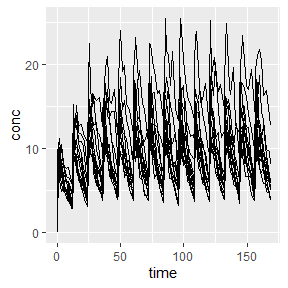# Noncompartmental evaluation of time to steady-state

#### 2023-04-29

Time to steady-state (TSS) can be estimated with PKNCA using either a monoexponential increase toward an asymptote or by a linear regression of the last points. According to Maganti (2008), the monoexponential method is preferred.

TSS can be estimated using either method using the pk.tss() function in PKNCA.

# Example

## Data setup

Illustrating time to steady-state, the example from the superposition vignette will be used.

library(PKNCA)
theoph_corrected <- as.data.frame(datasets::Theoph)
theoph_corrected$conc[theoph_corrected$Time == 0] <- 0
conc_obj <- PKNCAconc(theoph_corrected, conc~Time|Subject)
steady_state <- superposition(conc_obj, dose.times = seq(0, 168 - 12, by=12), tau=168, n.tau=1)
# Add some noise to the data so that it seems more reasonable
steady_state_noise$conc <- withr::with_seed( seed = 5, steady_state_noise$conc*exp(rnorm(nrow(steady_state_noise), mean = 0, sd = 0.1))
)

Examine the data graphically.

library(ggplot2)
ggplot(steady_state_noise, aes(x=time, y=conc, groups=Subject)) + geom_line()## Estimate time to Steady State

### Monoexponential

The below code estimates four different types of monoexponential time to steady-state:

1. tss.monoexponential.population: The population estimate of TSS using a nonlinear mixed effects model (one value for all subjects)
2. tss.monoexponential.popind: The individual estimate from a nonlinear mixed effects model (one value per subject)
3. tss.monoexponential.individual: The individual estimate using a gnls model to estimate each subject separately (one value per subject)
4. tss.monoexponential.single: The mean estimate of TSS using a nonlinear model
tss_mono <-
pk.tss.monoexponential(
conc = steady_state_noise$conc, time = steady_state_noise$time,
subject = steady_state_noise$Subject, time.dosing = seq(0, 168 - 12, by=12) ) #> Warning in nlme.formula(conc ~ ctrough.ss * (1 - exp(tss.constant * time/tss)), #> : Iteration 1, LME step: nlminb() did not converge (code = 1). Do increase #> 'msMaxIter'! #> Warning in nlme.formula(conc ~ ctrough.ss * (1 - exp(tss.constant * time/tss)), #> : Iteration 2, LME step: nlminb() did not converge (code = 1). Do increase #> 'msMaxIter'! tss_mono #> subject tss.monoexponential.population tss.monoexponential.popind #> 1 1 26.41707 36.94243 #> 2 10 26.41707 31.95582 #> 3 11 26.41707 22.79711 #> 4 12 26.41707 26.59872 #> 5 2 26.41707 23.53946 #> 6 3 26.41707 24.72254 #> 7 4 26.41707 25.73825 #> 8 5 26.41707 26.93414 #> 9 6 26.41707 23.21916 #> 10 7 26.41707 25.39759 #> 11 8 26.41707 24.54242 #> 12 9 26.41707 24.61720 #> tss.monoexponential.individual tss.monoexponential.single #> 1 40.65290 27.87592 #> 2 24.72591 27.87592 #> 3 20.18663 27.87592 #> 4 22.58610 27.87592 #> 5 25.45251 27.87592 #> 6 29.97015 27.87592 #> 7 21.60401 27.87592 #> 8 25.04023 27.87592 #> 9 23.53884 27.87592 #> 10 31.37369 27.87592 #> 11 32.02870 27.87592 #> 12 26.01334 27.87592 The fraction of steady-state required for time to steady-state can be changed with the tss.fraction argument (see ?pk.tss.monoexponential). ### Stepwise Linear The stepwise linear method estimates if the slope of the predose concentrations is statistically significant starting from the last measurement and moving backward in time. It has bias in that more individuals will move the time to steady-state to a late time point. tss_step <- pk.tss.stepwise.linear( conc = steady_state_noise$conc,
time = steady_state_noise$time, subject = steady_state_noise$Subject,
time.dosing = seq(0, 168 - 12, by=12)
)
tss_step
#>   tss.stepwise.linear
#> 1                 108